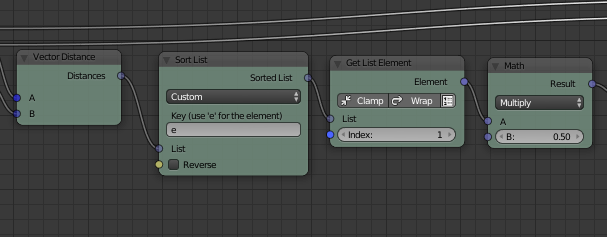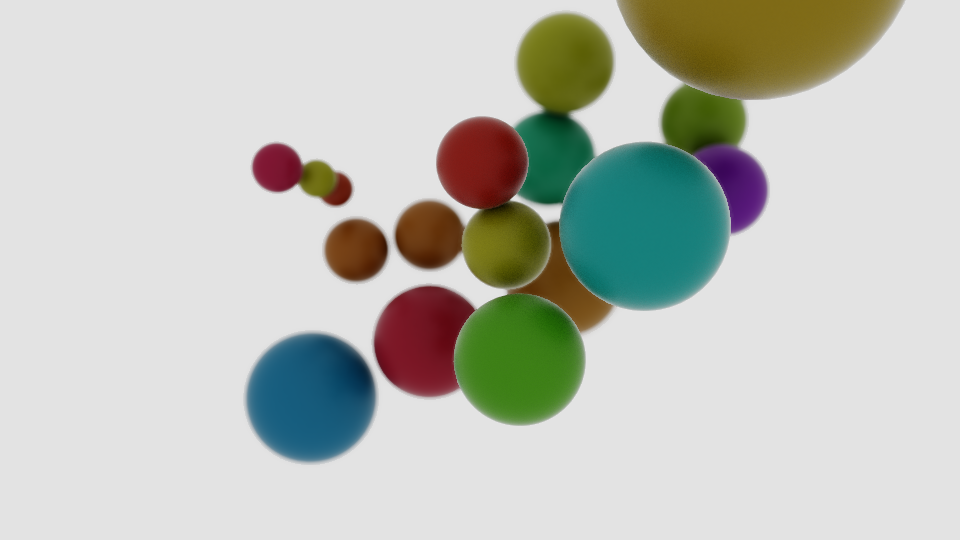AN Experiment 2018 Test 6 - dont overlap

For this animation nodes experiment I created a group of spheres and calculated their sizes so that they touch but never overlap. I iterated over the list of positions and calculated the distances to all other spheres using a Vector Distance Node. Then I sorted the list of distances and took the second entry to get the minimal distance to a different node (the first entry is always zero - its the distance of the current sphere to it self). The sphere is then scaled to half of the minimum distance to make sure no spheres overlapThe spheres are moved using a Vector Wiggle node